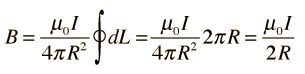Home » Ask Physics » calculating number of turns

# calculating number of turns

How to determine the number of turns needed to generate specific magnetic field?
my condition is that the area of the magnet field strength must be able to sense a magnet material which is placed 4meters away from it. And voltage applied is 2.6v. Is there any formula?

The question is not very clear. Is it a single loop whose area is to be determined or whether the area is given and no. of turns to be calculated?

Anyway, I am giving here the formulae.

If, V is given, then current can be calculated if R is given.Use this online calculator to solve your question, if you have necessary data.

http://hyperphysics.phy-astr.gsu.edu/hbase/magnetic/curloo.html

If any clarifications required please post as comment to this post.

This site uses Akismet to reduce spam. Learn how your comment data is processed.

### Hits so far @ AskPhysics

• 2,274,296 hits